# Evaluation

## Comparison of test data and CFD

### Introduction

All simulated cases are listed in Table 6, where the following abbreviations are used:

• Code – the computational code: Ansys Fluent (AF) or OpenFOAM (OF),
• M – mesh: according to Computational domain section above,
• N – convective schemes: the second-order upwind (SOU), the normalized variable diagram (NVD) (γ ), the total variation diminishing (TVD),
• CF – flow conditions: according to Table 1,
• TR – the approach for solution of the Navier-Stokes equations, (U)RANS , SAS or LES,
• TM – turbulence model: k-ε (SKE), k-ω SST (SST), k-equation eddy-viscosity sub-grid scale model (TKE), Smagorinsky (SMAG),
• TCM – turbulence-chemistry interaction model: Eddy Dissipation Concept (EDC), Turbulent Flame Closure (TFC),
• CH – chemistry mechanism: according to this section,
• R – radiation sub-model: P1 or none,
• Sct – turbulence Schmidt number,
• Prt – turbulence Prandtl number,
• Two and Twc – temperature boundary conditions for the obstacle and channel walls, respectively: zero-gradient (zg), isothermal (Tisoth = 300 K and Tisoth = 600 K for cases C1 and C2, respectively) or conjugate fluid-solid heat transfer (CHT).

For a quantitative validation of the present SAS and LES simulations, the averages have been obtained from the computational results by sampling over 40 vortex shedding periods (Nvs ) for the SAS non-reactive solution and three flow-through times for the combustion SAS and LES. The flow-through time was defined as the ratio between the axial length of the computational domain to the jet bulk velocity.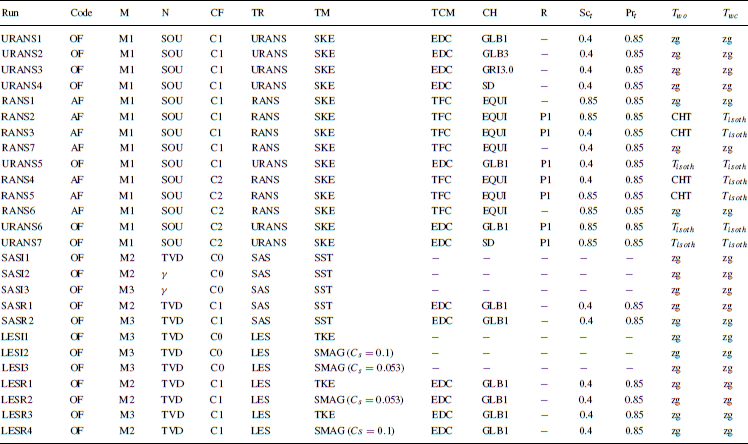Table 6: Run matrix for the Volvo test rig.

### Inert calculations (SAS,URANS)

Figure 9 shows the measured and predicted mean stream-wise velocity and its fluctuation as well as the normalized turbulence kinetic energy along the central-line behind the obstacle (for case C0). For the sake of completeness, besides the LDA data by Sjunnesson et al. , the LDV data by Sanquer et al. , who had investigated inert bluff-body wakes as well as premixed bluff-body combustion, were added to the plot. The experimental data by Sanquer et al.  have been obtained for the Reynolds number, Re ≈ 6×103, based on the bluff body height (case I3). Another important parameter in this experiment, the blockage ratio (the ratio between bluff-body to channel heights) was 0.33 as in the Volvo test rig.

Apart for the present SAS calculations, the author’s previous URANS results  obtained with the low-Reynolds-number k-ε turbulence model of Launder and Sharma were included for comparison, as well as LES and DES results published by Hasse et al. . Three inert SAS calculations have been carried out. SASI1 and SASI2 cases differed only by the applied discretization schemes for the convective terms, TVD vs. NVD. The SASI3 case was calculated using the Gamma scheme as well, to check the influence of the grid resolution. In their computations, Hasse et al.  utilized the Ansys CFX solver and the CDS-2 scheme for LES, and a bounded second order upwind biased discretization scheme for DES.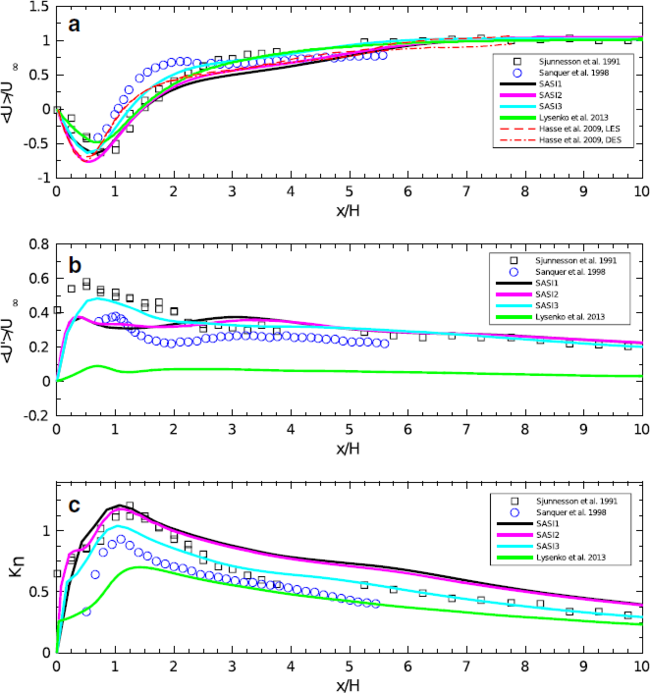Figure 9: Normalized mean stream-wise velocity (a), its fluctuations (b) and and normalized turbulence kinetic energy (c) in the wake centerline for the Volvo test rig , case C0

Overall, there is a reasonable match between numerical and experimental data. One can observe the same trends between all numerical runs for the axial distribution of the mean stream-wise velocity. For the stream-wise RMS velocity the SASI3 case provided the best result. The normalized turbulence kinetic energy, Kn = √k/U, where the turbulence kinetic energy k = 3/4(u’2 + v’2), is shown in Fig. 9c. Results from SASI1 and SASI2 predicted quite well the measured turbulence kinetic energy in the near wake (up to x/H = 2), while over-predicting it afterwards. In contrast, the SASI3 calculations matched well the kinetic turbulence energy in the far wake, while under-predicting it in the near wake. The difference between measurements and numerical calculations for the mean recirculation zone length <Lr> is significant and often subject to discussion. By definition, the recirculation length <Lr> corresponds to the distance between the base of the triangular cylinder and the sign change of the centerline mean stream-wise velocity. The quality of <Lr> predictions may be considered as the deciding factor for the agreement between the experimental and numerical results. Some discrepancies were observed between mean velocities inside the recirculation zone. These deviations can be affected by the earlier laminar-turbulent transition in the separating shear layers, but also partially due to a lack of statistical convergence. In the present SAS, the recirculation zone length was predicted as <Lr>/H = 1.4 for the SASI1 and SASI2 runs, which is in fairly good agreement with experimental data of Sjunnesson et al. , <Lr>/H = 1.35. It is interesting that Hasse et al.  predicted the recirculation lengths with the LES and DES models similar to the present SAS results (<Lr>/H = 1.18), which deviated only about 11% from the experimental measurement (<Lr>/H = 1.33).

Figure 10 shows the turbulent structures for this flow using the Q-criterion, (Q =S2 - ω2 = 5×104, where S is the strain rate and ω is the vorticity). The dynamics of the downstream flow behind the bluff-body was largely driven by the shear layer and wake processes alone. For this Reynolds number range (sub-critical flow regime, 1000 < Re < 200000), both absolute and convective instabilities are present – asymmetric vortex shedding (the Bénard/von Kármán instability) and Kelvin-Helmholtz instability of the separated shear layer . Investigation of the shear-layer instability was out of scope of the present work. The vortex shedding instability was periodic and had a characteristic frequency of fvs = StU/H, where St is the Strouhal number. As a general observation for circular cylinders, the Strouhal number is independent of Reynolds number (St = 0.21) in the post-shear-layer region. The Strouhal numbers determined from the Fast Fourier transform of the sampled velocity (about 1.8×105 samples during 40 vortex-shedding periods) were St = 0.28, 0.27 and 0.30 for the SASI1, SASI2 and SASI3 runs, respectively. Results obtained by URANS (with the low-Reynolds k-ε turbulence model of Launder and Sharma) and reported previously  were St = 0.28. This value was in reasonable agreement with the experimental data by Sjunnesson et al.  and Sanquer et al. , who measured St = 0.25 and St = 0.26, respectively. This corresponded with the LES data reported by Manickam et al.  (St = 0.28) and the Detached-Eddy Simulation by Hasse et al.  (St = 0.28) for the same Volvo configuration and identical conditions.

Figure 11 compares one-dimensional frequency spectra extracted from the present solutions at the downstream location x/H = 1.75 on the centerline of the wake. About 1.8×105 samples of the cross-flow velocities were collected (or Nvp ≈ 35). The spectra calculated from these time series were then averaged in the span-wise direction to increase the statistical meaning. To obtain the spectra, the Welch periodogram technique was used. The frequency was non-dimensionalized by the Strouhal shedding frequency (fvs). The spectra obtained by LES of Manickam et al.  and measured by Sanquer et al.  were added to compare the present SAS results with other numerical solutions. A −5/3 slope is shown as well. All numerical data sets yielded very similar power spectra. However, it is clearly seen that the numerical solutions provided over-dissipative spectra in respect to the −5/3 slope. It is worth noting that the present results were less dissipative than the LES data by Manickam et al.  and reproduced the same trend as the measured spectrum by Sanquer et al. . It is well-known that the effect of an excessive dissipation of a numerical method leads to a rapid decay of the spectrum so that no inertial subrange can be satisfactorily captured. The over-dissipation of the present method could probably be explained by using the TVD scheme to approximate convective terms in the momentum equation, which is more dissipative compared to CDS-2. Additionally, the unstructured hexahedral/tetrahedral mesh designed for the present calculations might have added extra dissipation to the method as well. And, of course, the implemented procedure for the high frequency damping of the turbulent viscosity using the WALE sub-grid scale model may also contribute.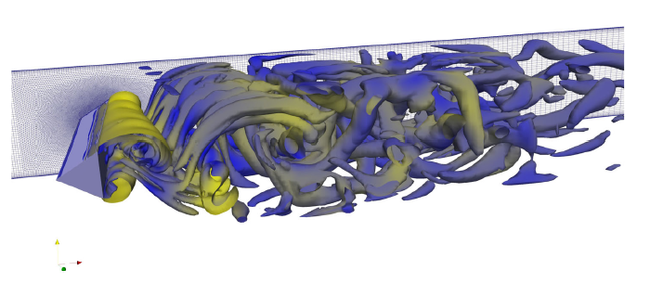Figure 10: Flow structures for the Volvo test rig, case C0. Iso-surface of the Q-criterion, Q = 5×104 (SAS2)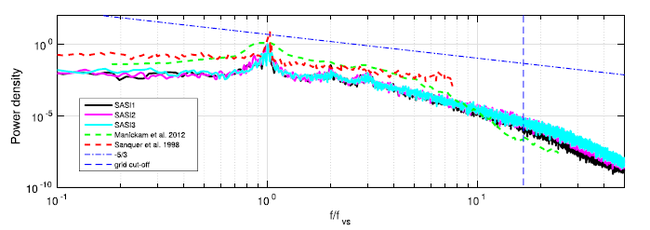Figure 11: One-dimensional spectra of the transverse velocity in the wake for the Volvo test rig: non reactive SAS results, case C0

### Inert calculations (LES)

Three non-reacting LES runs have been carried out to investigate the effects of the SGS models. For this purpose the k-equation and the Smagorinsky model with two different constants (Cs = 0.1 and Cs = 0.053, respectively ) were applied. For a quantitative validation of the present LES simulations, the averages have been computed by sampling over 50 vortex shedding periods (Nvs).

Figure 12 shows the measured and predicted mean stream-wise velocity and its fluctuation as well as the normalized turbulence kinetic energy along the center-line behind the obstacle. In general, all three LES runs match the experimental data by Sjunnesson et al.  reasonably well. The discrepancies between all SGS models are negligible. The differences between the LES and the SASI3 results are small as well. The recirculation zone length was predicted as <Lr>/H = 1.28 for all LES runs (the same as for SASI3), which is in fairly good agreement with experimental data of Sjunnesson et al. , <Lr>/H = 1.35.

Figure 13 compares one-dimensional frequency spectra extracted from the present solutions at the downstream location x/H = 1.75 on the centerline of the wake. About 6×105 samples of the cross-flow velocities were collected (or Nvp ≈ 50). For sake of completeness, the spectrum obtained by SASI3 was added to assess the dissipative properties of the SAS and LES results. It can be seen clearly that the spectra obtained by LES collapsed well and had a similar distribution, while the SAS spectrum became more dissipative after f/fvs = 2.5. The Strouhal numbers are St = 0.27, 0.29 and 0.28 for the LESI1, LESI2 and LESI3 runs, respectively. These values were in reasonable agreement with the experimental data by Sjunnesson et al.  and Sanquer et al. , who measured St = 0.25 and St = 0.26, and corresponded also well with the SAS runs.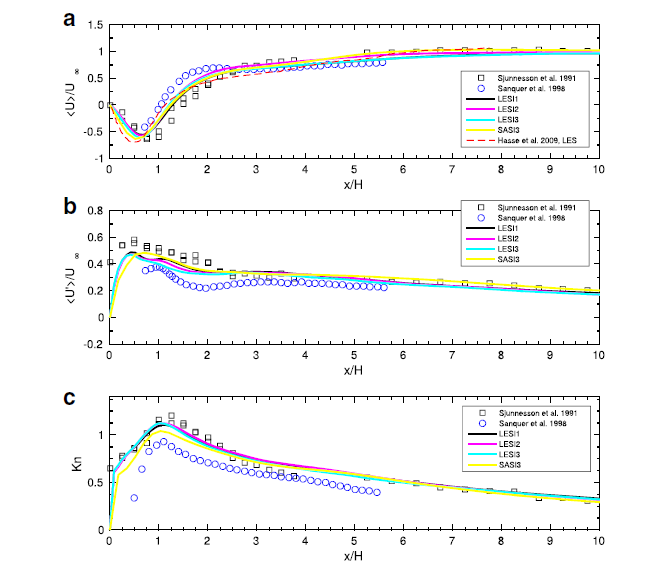Figure 12: Normalized mean stream-wise velocity (a), its fluctuations (b) and normalized turbulence kinetic energy (c) in the wake centerline for the Volvo test rig, case C0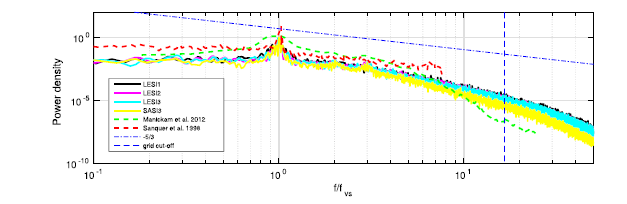Figure 13: One-dimensional spectra of the transverse velocity in the wake for the Volvo test rig: non reactive LES results, case C0

In conclusion, satisfactory agreement was obtained between the measured and calculated stream-wise velocity and turbulence kinetic energy by the present SAS and LES , which indicates, at least qualitatively, the adequate spatial resolution of the used grids.

### Reactive results (RANS/URANS)

Here, some limited RANS/URANS results obtained using the EDC and TFC combustion models are presented. First, the effects of heat transfer were studied. For this purpose, several calculations with conjugate (coupled fluid/solid interaction) heat transfer were carried out. Also, the influence of the turbulent Schmidt number was investigated. Thereafter, the effects of the finite-rate chemistry were investigated using both global and detailed mechanisms. Finally, the model sensitivity to the operational conditions was provided.

It is worth noting that the conventional RANS and unsteady-RANS approaches were used for the present calculations. The reactive RANS-TFC calculations obtained by AF were fully converged to the steady state solution without any artificial, numerical oscillations in the bluff-body wake. The main reason why the URANS approach was adopted for OF was difficulties to get stable and converged flow in the bluff-body wake. This is probably due to the fact that the OF solver(s) and applied schemes are less numerically dissipative compared to AF. Vice versa, the authors were not able to get any unsteadiness in the wake using the URANS-TFC approach in AF. Additionally, it was observed that the URANS-EDC approach by OF provides slightly more accurate results compared to RANS-TFC by taking into account some dynamic effects in the bluff-body wake.

#### Heat transfer effects

Several RANS/URANS calculations were performed to investigate the influence of the heat transfer effects. For this purpose, the two-dimensional mesh was re-designed to calculate the conjugate (fluid/solid) heat transfer. A new computational zone was added to the mesh, which included the solid bluff body. Figure 14 displays contours of temperature (cases C1 and C2). The combustion RANS was performed using the Ansys Fluent code. The premixed combustion was treated using the TFC model. The first run (RANS1) was calculated using adiabatic conditions for all walls and excluded radiation. The second run (RANS2) included the conjugate and radiative (P1) heat transfer. The standard k-ε model was used for the turbulence closure for both cases. The third run (RANS3) was conducted to estimate the influence of the turbulent Schmidt number, which here was set to Sct = 0.4. The last run (RANS4) was similar to RANS3, but was calculated for the case C2. As has been discussed in the literature [20, 21, 22], the values of Sct = 0.3 – 0.4 rather than the more widely used values of 0.7 – 0.9 can provide better agreement for several of the considered test cases. Afterwards, the temperature on the wall surfaces of the bluff-body was extracted and then applied as boundary condition to the standard mesh in order to estimate the heat transfer effects for the EDC model (URANS5).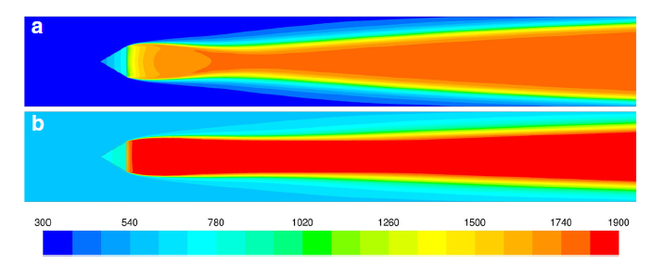Figure 14: Contours of mean temperature for the Volvo test rig for the case C1 (a) and the C2 (b), obtained by RANS2 and RANS4, respectively

Figure 15 displays a comparison of the mean stream-wise velocity at several axial positions of the channel downstream of the bluff-body. In general, agreement between calculated and experimental results inside the recirculation zone (x/H = 1.525) is reasonable. The largest deviations can be observed to occurr in the recovery region at x/H = 3.75 and x/H = 9.4, which could be explained by the fact that all runs significantly underestimate the recirculation zone length. All runs (except RANS1) predicted approximately the same recirculation zone length of Lr = 1.8, which is significantly lower compared to the experimental value Lr,exp = 3.55. It is interesting that both EDC and TFC models provided qualitatively similar results. The difference between RANS1 and RANS2 is seen clearly as well. RANS1 provided more rapid flame brush expansion resulting in the shortest recirculation zone length.

Figure 16 shows distributions of the mean mole fractions of XCO2, XO2 and temperature for the present calculations and the experimental results. The effects of heat transfer (RANS1 vs. RANS2) were a minimum at x/H = 3.75 and increased significantly further downstream. The distributions of mean scalars show the same trends as the flame structure. The numerical results obtained by the EDC (URANS5) and TFC (RANS3) models agree quite well with each other, in spite of small deviations for the mean temperature, where the EDC model slightly over-estimated the TFC results. Also, it is clearly seen that calculations obtained using Sct = 0.4 are in better agreement with the experiments than the results with Sct = 0.85, consistent with the velocity field.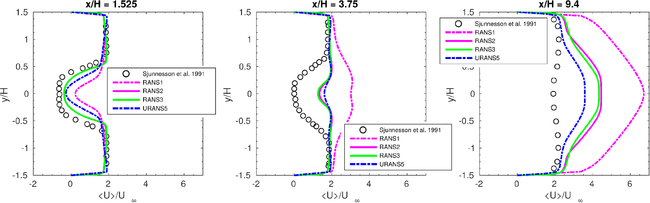Figure 15: Mean profiles of the normalized stream-wise velocity, obtained by RANS1, RANS2, RANS3 and URANS5 for the Volvo test rig, Case C1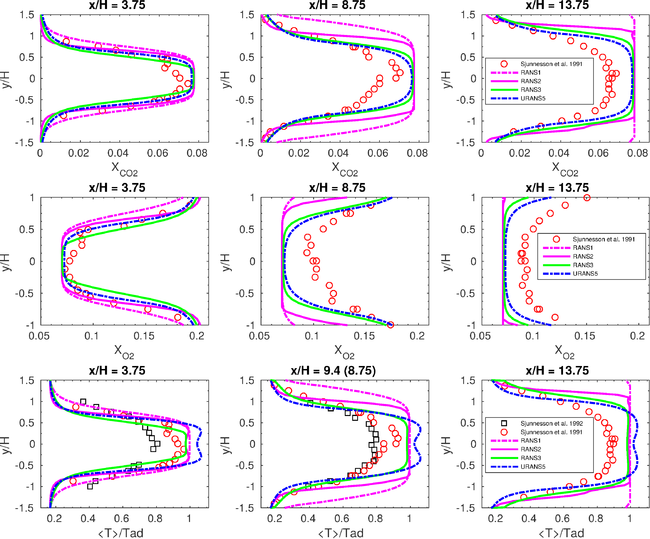Figure 16: Transverse profiles of mean mole fractions XCO2, XO2 and temperature, obtained by RANS1,RANS2, RANS3 and URANS5 for the Volvo test rig, Case C1

#### Finite-rate chemistry effects

Several chemical kinetics schemes were chosen to investigate the finite-rate chemistry effects: global one-step (GL1) and three-step (GL3) as well as the full GRI-3.0 (GRI3.0) and San-Diego (SD) mechanisms. Figure 17 compares the experimental data with the present results. In general, temperature profiles predicted by the EDC with different kinetics schemes matched the experimental data quite well. The EDC with all the kinetic schemes over-predicted the peak temperatures by about 150 K. However, this deviation can be explained by the simplified treatment of heat transfer effects near the solid walls (adiabatic conditions) and by the fact that the radiation losses were not taken into account. Some deviations between all kinetics schemes were observed for the mole fraction of carbon dioxide as well as for the mole fraction of oxygen, O2.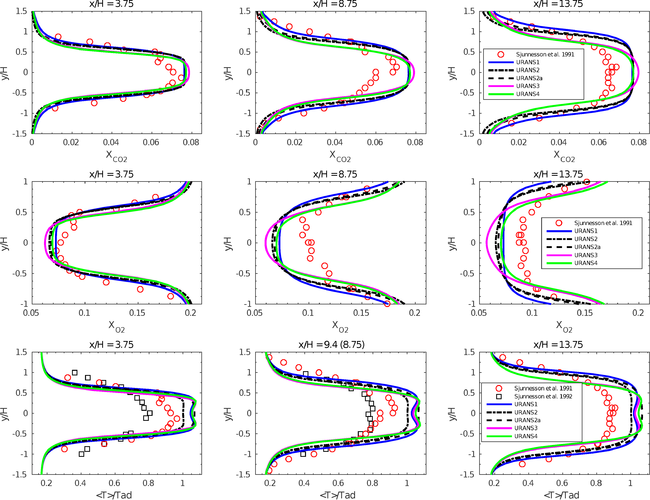Figure 17: Mean profiles of mean mole fractions XCO2 , XO2 and temperature for the Volvo test rig, case C1

#### Effects of operational conditions

Several RANS and URANS calculations using both the TFC and EDC models were performed to investigate the models’ sensitivity to the different operational conditions. For this purpose, the second baseline case (C2 from Table 1) from the series of experiments by Sjunnesson et al. [1-3] was chosen. It is worth noting that all present calculations were carried out for the equivalence ratio φ = 0.65, while the CARS measurements have been obtained for the significantly lower value, φ = 0.58. RANS4 and RANS5 were calculated using the TFC model and the same settings as RANS3 and RANS2. The only difference between RANS4 and RANS5 was the value of the turbulent Schmidt number, Sct = 0.4 and Sct = 0.85, respectively. Figure 14b shows contours of the temperature obtained by RANS4. URANS6 and URANS7 were performed using the EDC model with different treatment of chemical kinetics, namely the global one-step reaction and the full San-Diego mechanism, respectively. The boundary conditions for the temperature on the bluff-body walls were interpolated from the data extracted from RANS5. The turbulent Schmidt number was set to Sct = 0.85 for both URANS6 and URANS7, based on results obtained by the TFC model.

Figure 18 shows comparisons of the mean molar fractions of XCO2, XO2 and temperature at x/H = 3.75, 8.75 and 13.75 between the present calculations and experimental results. At x/H = 3.75, satisfactory agreement was obtained between both experimental profiles and all four numerical runs with some small differences of about 5% and 10% for the peak temperatures and mole fractions, respectively. At x/H = 8.75, considerable deviations of about 20% were observed for both mole fractions for all four runs. At the same location, the measured temperature was matched quite well by the EDC runs (URANS6 and URANS7), while the TFC runs (RANS4 and RANS5) provided satisfactory agreement with deviations of 10%. Further downstream, at x/H = 13.75, reasonable agreement (with deviations of about 10%) was observed for both mole fractions and temperature for all runs inside the flame. However, significant differences could be observed at the channel walls, where all numerical runs under-predicted the temperature (and mole fractions) at the channel walls. These discrepancies might be explained by the applied iso-thermal boundary conditions, which can be inaccurate for the flame-wall interaction.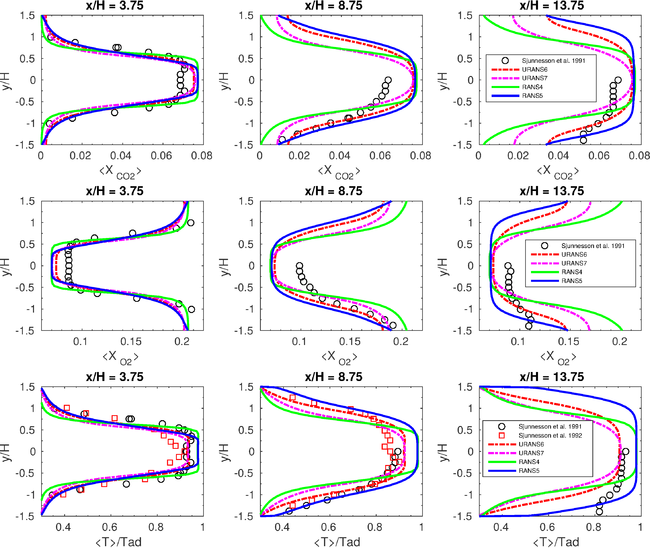Figure 18: Transverse profiles of scalar data obtained by RANS4, RANS5, URANS6 and URANS7 for the Volvo test rig, case C2

### Reactive results (SAS, LES)

The mean stream-wise velocity <u> and the mean turbulence kinetic energy <Kn> along the centerline are shown in Fig. 19. One can clearly see that the LES results match the experimental data by Sjunnesson et al.  with reasonable agreement as well as the experimental data by Sanquer et al.  (Case r3). The differences between the Smagorinsky and the k-equation SGS models are insignificant. Some discrepancies can be observed in the vicinity of the bluff body inside the recirculation zone, which could be explained by the simplified treatment of the heat transfer effects. The SAS results revealed significant under-prediction of the recirculation zone length, which could possibly be explained by, besides the effects of heat transfer, the RANS treatment of the turbulence-chemistry interaction. Indeed, the SAS results for the mean stream and transverse velocities were quite similar to those provided by the RANS/URANS calculations. The axial distribution of <Kn> has the same trend as that of the stream velocities. However, it is interesting to note that the Smagorinsky model (LESR2) provided significantly higher levels of the turbulence kinetic energy. Such over-estimation could, possibly, be explained by the setting of the Smagorinsky constant. Manickham et al.  and Ma et al.  have applied Cs = 0.1 in their reactive simulations of the Volvo rig. Furthermore, as was discussed by Ma et al. , the Smagorinsky model is known to be sensitive to the level of heat release. In the present study, to avoid excessive dissipation, the Smagorinsky constant, Cs, was set to Cs = 0.053, which is slightly lower than the conventional minimum limit Cs = 0.065.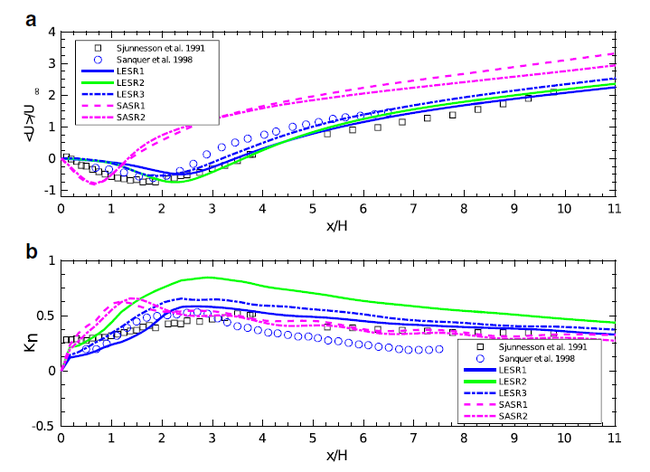Figure 19: Mean profiles of the normalized axial velocity (a) and the turbulence kinetic energy (b) obtained by the reactive SAS and LES models for the Volvo test rig, case C1

The mean recirculation zone lengths were predicted by SAS and LES as <Lr>/H = 1.36 – 1.53 and <Lr>/H = 3.23 – 3.75, respectively. The experimental values measured by Sjunnesson et al.  and Sanquer et al.  were Lr,exp/H = 3.34 and Lr,exp/H = 2.88, respectively.

Figure 20 presents distributions of the normalized mean stream-wise velocity and its fluctuation at several axial positions in the channel downstream of the flame-holder. The agreement between the predicted and measured <u> obtained by the LES models is reasonable, while the SAS results show fairly large deviations for the velocity field and the recirculation zone length, leading to greater axial flow acceleration in the wake. Inside the recirculation zone, the LES models failed to reproduce the measured profile of the mean stream-wise velocity fluctuations. One possible explanation for these discrepancies could be insufficient grid resolution of the separated shear layers. Outside the recirculation zone, reasonable agreement can be observed for the k-equation SGS model, whereas the Smagorinsky model over-estimated the values of <u’> according to Fig. 19b. The SAS model predicted the mean stream-wise fluctuating velocity reasonably well inside the recirculation zone. Outside of the recirculation zone, significant under-estimation can be observed.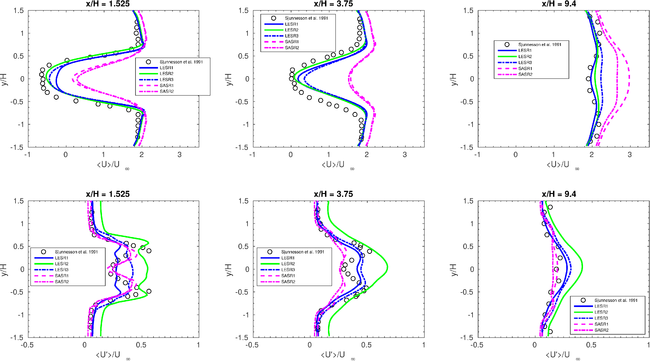Figure 20: Mean profiles of the normalized stream-wise velocity and its fluctuations obtained by the reactive SAS and LES models for the Volvo test rig, case C1

Instantaneous flame visualizations of the predictions by the SAS and LES models using the Q-criterion are shown in Figs. 21 and 22. Both SAS and LES displayed symmetrical flame propagation or symmetrical vortex shedding, which was observed experimentally with high-speed video and Schlieren imaging . As expected, the flow structures obtained by the LES runs contained larger amounts of small-scale structures clearly corresponding to the difference between the SAS and LES approaches. The principle difference between LES and SAS approaches resulted in a wider and less wrinkled flame obtained by the SAS runs. Another interesting observation following from Fig. 22 is that the LESR2 run predicted a staggered pattern due to vortex-vortex and vortex-flame interactions.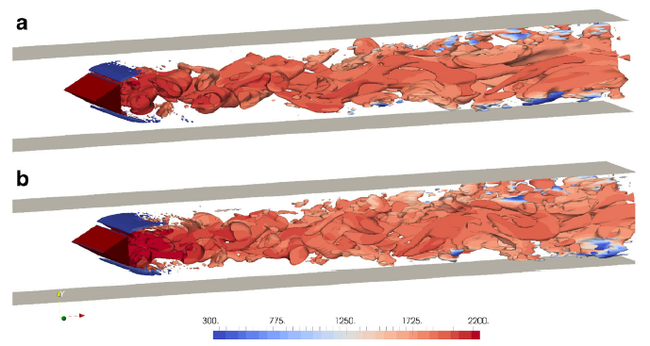Figure 21: Flow structures obtained by the reactive SASR1 (a) and SASR2 (b) for the Volvo test rig, Case C1. Iso-surface of the Q-criterion (Q = S2 - Ω2 = 1×104, where S is the strain rate and Ω is the vorticity) colored according to the temperature [K]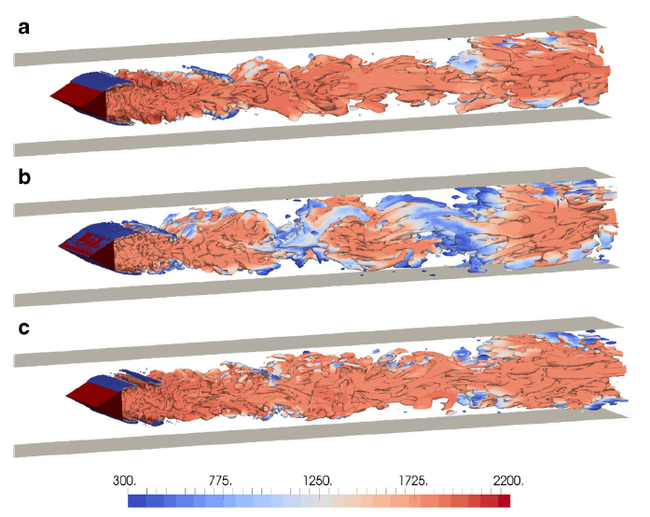Figure 22: Flow structures obtained by the reactive LESR1 (a), LESR2 (b) and LESR3 (c) for the Volvo test rig, case C1. Iso-surface of the Q-criterion (Q = S2 - Ω2 = 1×104, where S is the strain rate and Ω is the vorticity) colored according to the temperature [K]

The bluff-body combustion process is characterized by an existence of large vortex structures and their shedding in the near wake of the triangular cylinder, where the convective Kelvin-Helmholtz instabilities play a dominant role. In the present study, the vortex shedding frequencies were predicted as fvs = 201 Hz and fvs = 140 – 165 Hz by SAS and LES models, respectively. Figure 23 displays turbulent energy spectra extracted from the present LES and SAS solutions at the downstream location x/H = 1.75 on the centerline of the wake. The frequencies in Fig. 23 were normalized by the relevant vortex shedding frequency. For the sake of completeness, the reactive LES results by Manickam et al.  were added, in spite of the fact that these results were calculated for the C2 case, as well as the measured spectrum by Sanquer et al. . The spectra calculated by the LES and SAS models displayed clearly a −5/3 region. The spectra by all LES runs compared well with the spectrum measured by Sanquer et al.  and showed the similarity of the coherent structures in the reactive wake. It can be seen clearly that the SAS results provide more dissipative spectra compared to the LES runs. It is interesting that the spectra by SAS-EDC were quite similar to the spectra reported by Manickam et al. .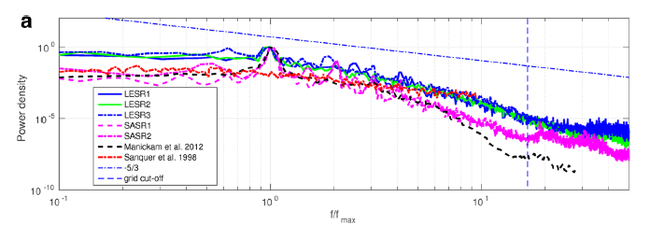Figure 23: Fourier power spectra (a) of the transverse velocity in the wake for the Volvo test rig: reactive SAS and LES results, case C1

Figures 24 and 25 compare the CARS temperature measurements  and the gas analysis data  with the numerically predicted results respectively. Note that the experimental data by CARS and gas analysis have been collected at different positions (x/H = 9.4 for CARS and x/H = 8.75 for gas analysis). It should also be noted that the temperature from the gas analysis data was computed from the measured species, assuming equilibrium chemistry.

Both LES and SAS runs failed to predict the temperature inside the recirculation zone (location x/H = 0.95), which could possibly be explained by the fact that heat transfer effects were not taken into account. Outside the recirculation zone, satisfactory agreement between LES and SAS runs was observed. The LESR2 run, with the Smagorinsky SGS model, provided the most accurate predictions, while the LESR1 and LESR3 runs, with the k-equation model, slightly over-estimated the temperature profiles. SAS results predicted a more sharpened distribution of the mean temperature profiles and over predicted the temperature (due to the wider and less wrinkled flame structure), compared to the experimental data as well as to the LES results. In terms of temperature fluctuations, LES produced quantitatively and qualitatively distributions similar to the experimental ones. However, the Smagorinsky model has the trend to over-estimate the pulsations in the flame core, while the k-equation model resulted in its under-estimation. The SAS failed to reproduce the temperature fluctuations in the flame core even qualitatively. Several transverse distributions of the mean mole fractions XCO2 and XO2 are shown in Fig. 25.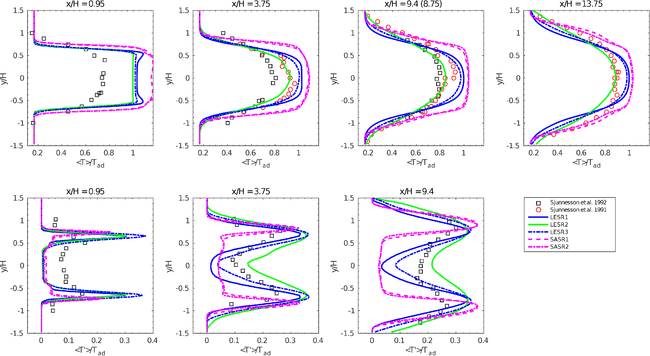Figure 24: Transverse profiles of normalized mean temperature and its fluctuations obtained by the reactive SAS and LES models for the Volvo test rig , Case 1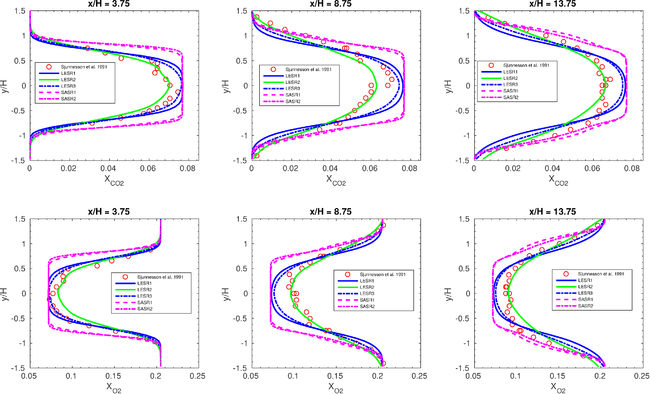Figure 25: Transverse profiles of the mole fractions XCO2 and XO2 obtained by the reactive SAS and LES models for the Volvo test rig, Case 1

Contributed by: D.A. Lysenko and M. Donskov — 3DMSimtek AS, Sandnes, Norway